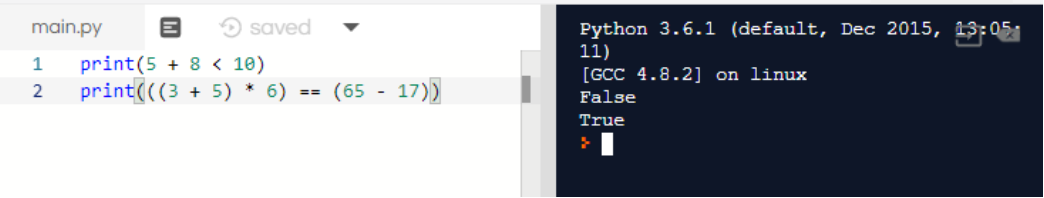# Booleans

Booleans are True or False statements. Unlike strings or numbers, booleans store statements of truth: is what I’m saying true or false?

For example, if I say, “You are a robot”, a boolean can store whether this statement is true. In this case, since you are not a robot (hopefully!), False would be stored.

1. I am a human. _______
2. I have 25 fingers. _______
4. My favorite color is blue. ______

The most common forms of boolean operators are comparisons like less than or greater than. How these are written in python are listed below.

OperatorDescriptionOperatorDescription
<Less than>Greater than
<=Less than or equal to>=Greater than or equal to
==Equal to!=Not equal to

### Challenge 1

As usual, use print to print out your results to the following:

print(5 + 8 < 10)
print((3 + 5) * 6) == (65 - 17)


The first statement should return False. And the second should return True.### Challenge 2

Try printing out the answers to the following expressions using print. If the results for any of these statements don’t make sense, please ask for help!

• 54 < (10 + 32)
• (37 / 5) != 7
• “Hello” + “World” == “Hello World”
• False == False

### Fun Fact: Connecting Booleans with AND and OR operators

You can also connect boolean expressions together using the AND and the OR operator. Here’s a chart that describes what happens when we connect booleans together:

ExpressionResult
True and TrueTrue
True and FalseFalse
False and TrueFalse
False and FalseFalse
True or TrueTrue
True or FalseTrue
False or TrueTrue
False and FalseFalse

To summarize, AND requires both boolean expressions to be true, while OR only requires one of the two Boolean expressions to be true. Here are some more examples:

• (5 < 8) and (9 != 10) produces True since both 5 is less than 8 and 9 is not equal to 10.
• (8 <= 2) or (“h” + “e” == “he”) produces True since “h” + “e” results in “he”, even though 8 is not less than or equal to 2.
• (6 != 2 * 3) or (8 < 2 * 4) produces False since both 6 not equal to 2 * 3, and 8 being less than 2 * 4, produce False.

#### What do you think the following expressions produce?

• (11-2 < 10) and (7+3 > 10)
• (True or 3 < 1) and (False or 3>1)